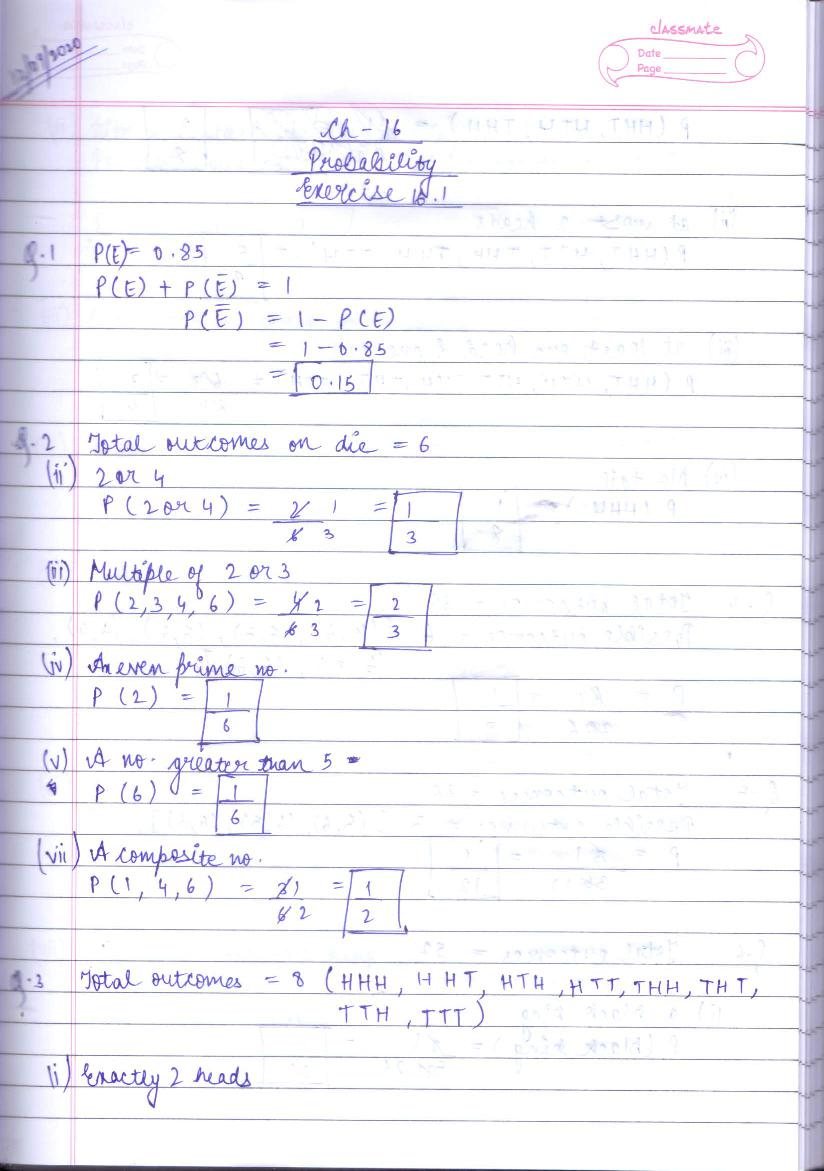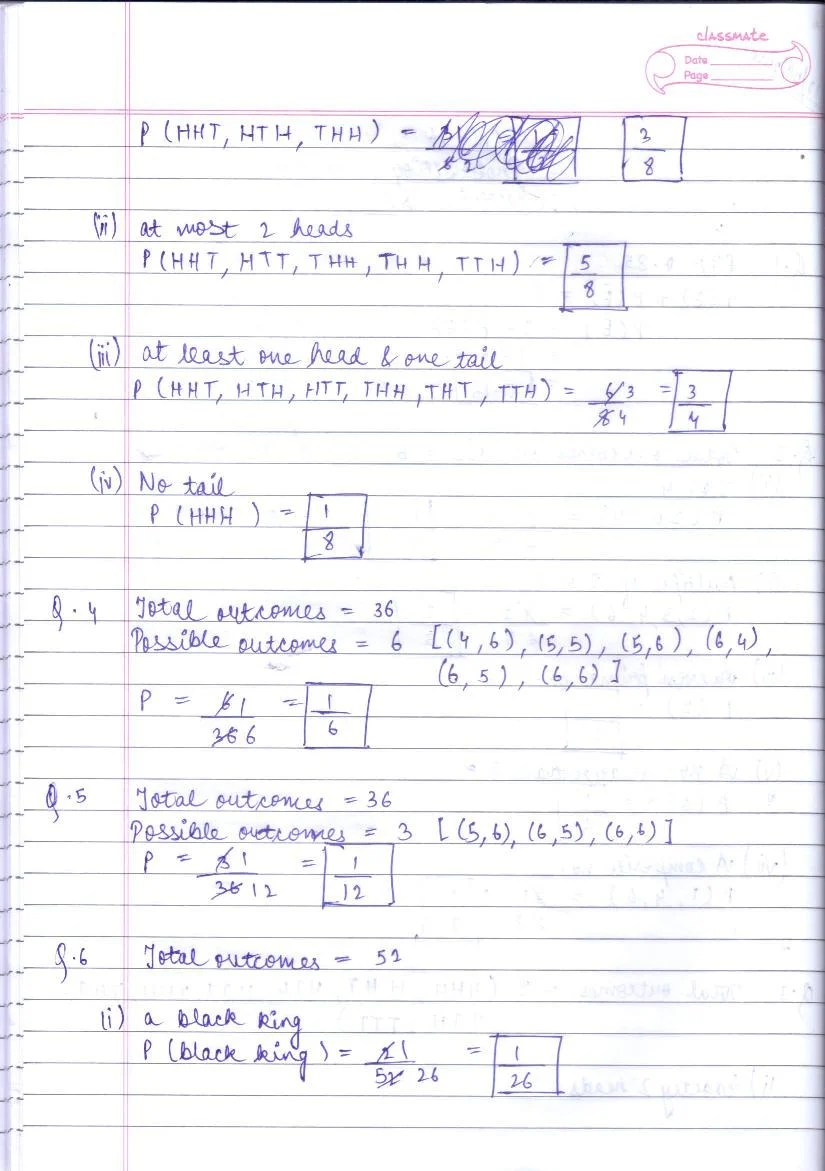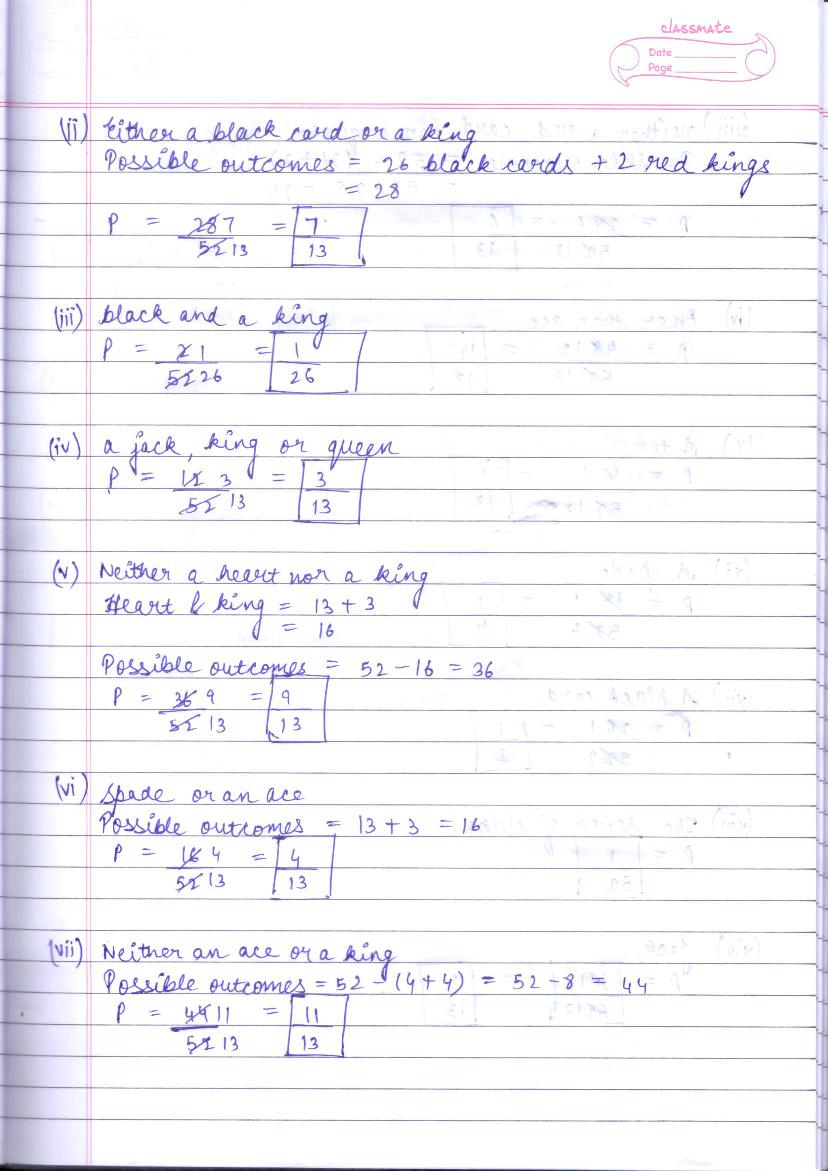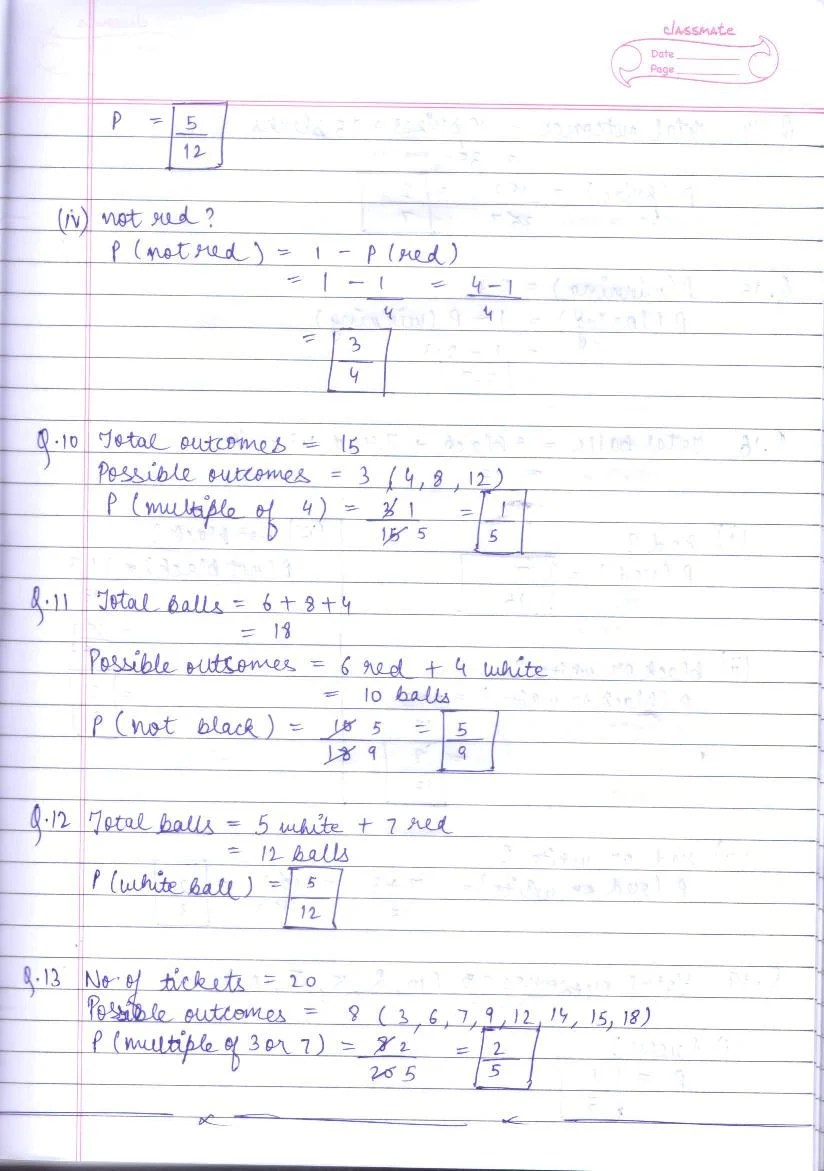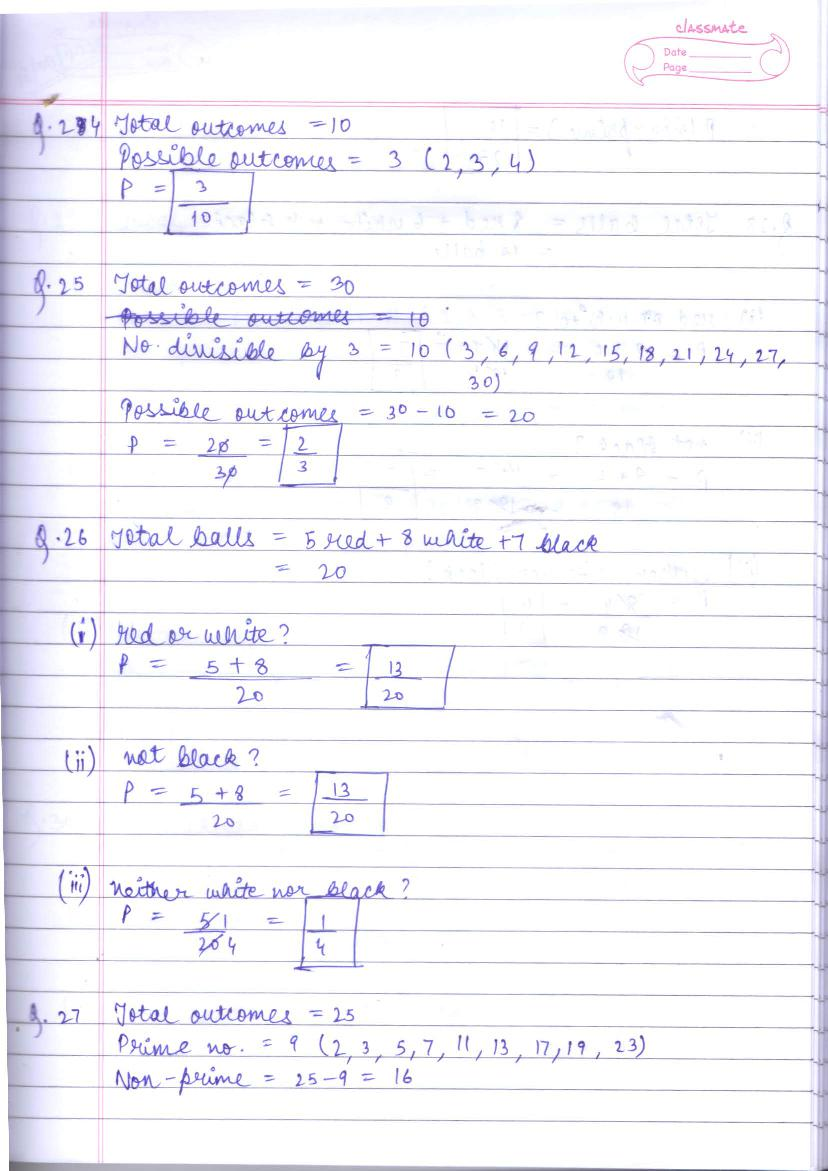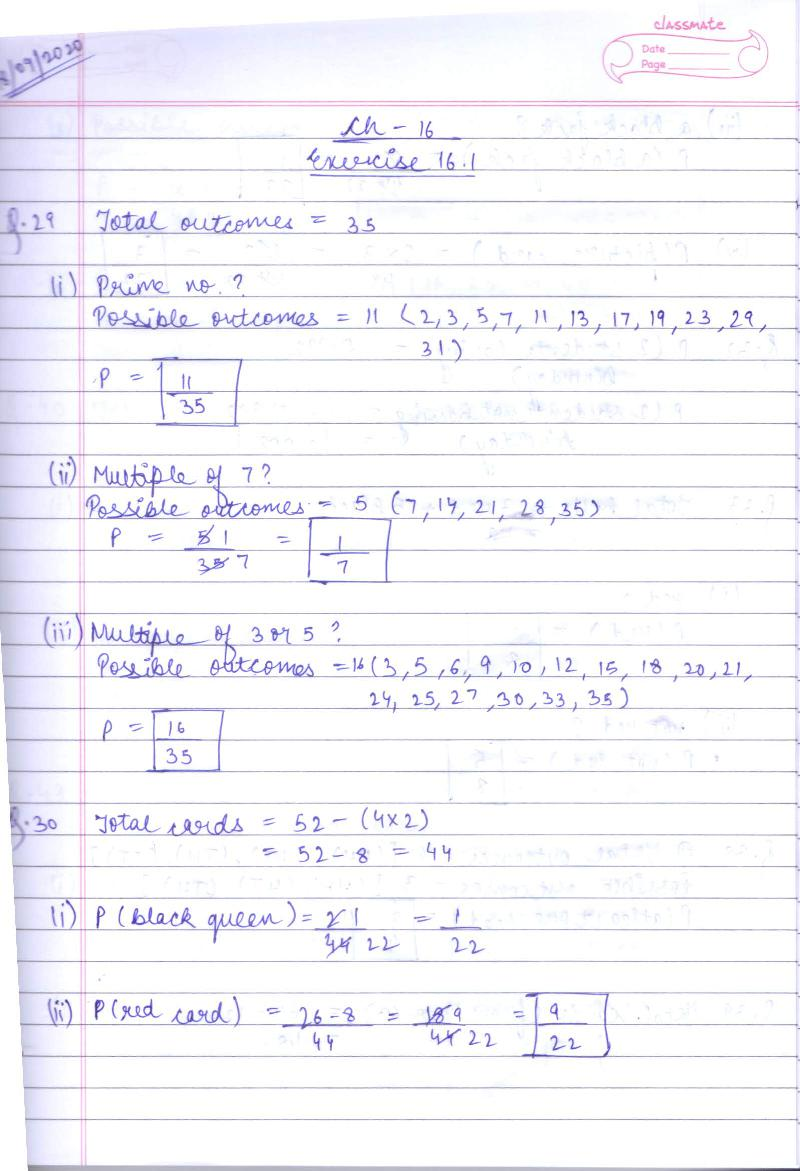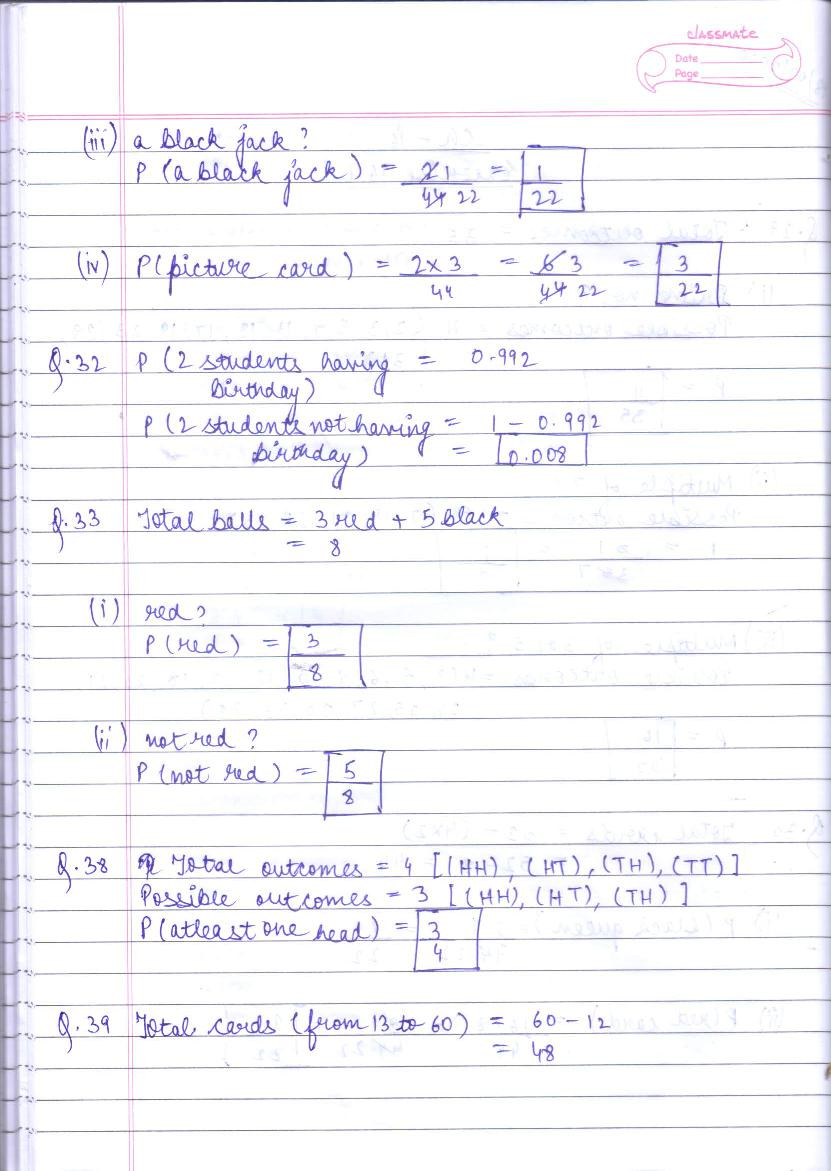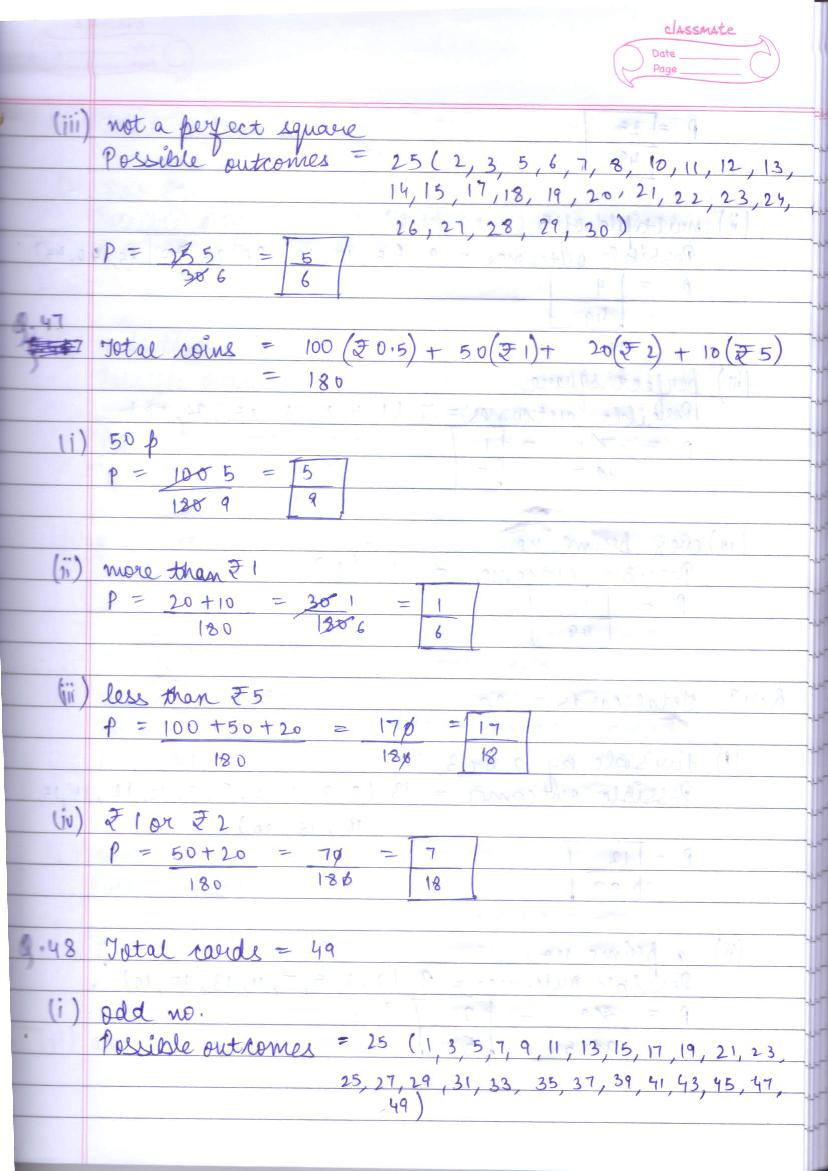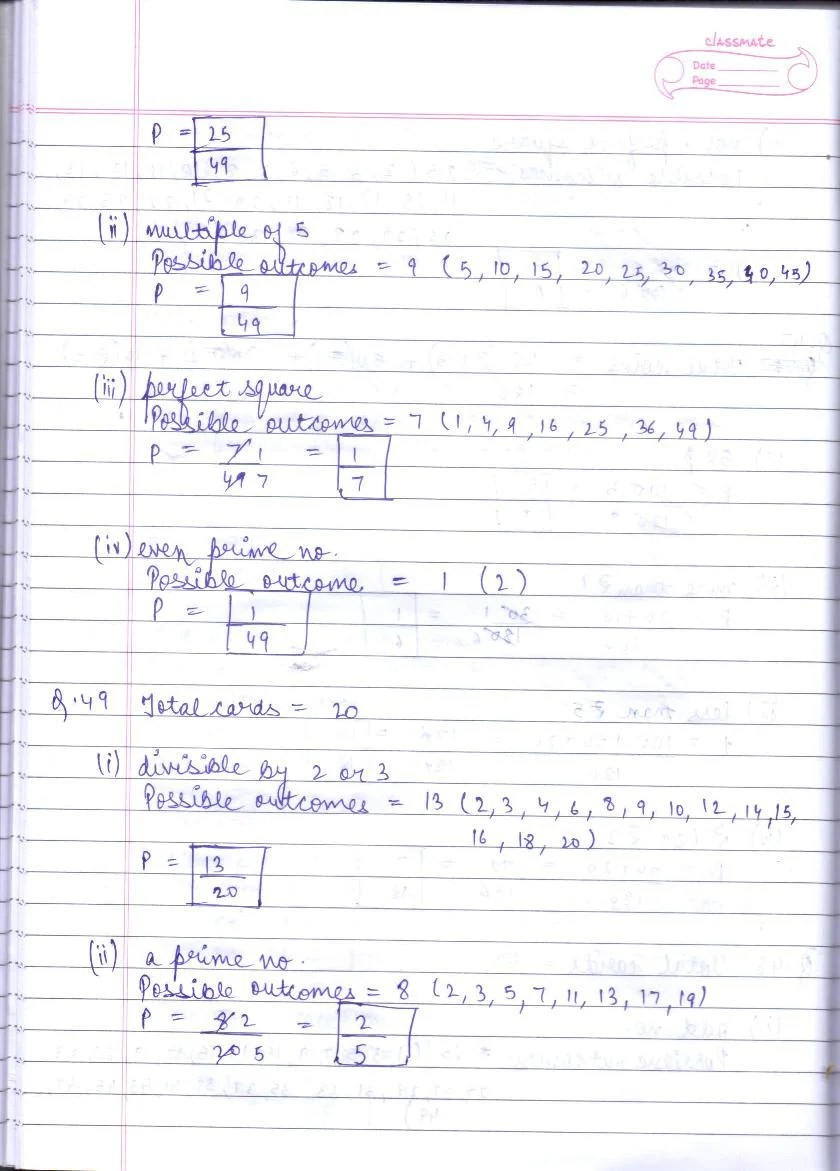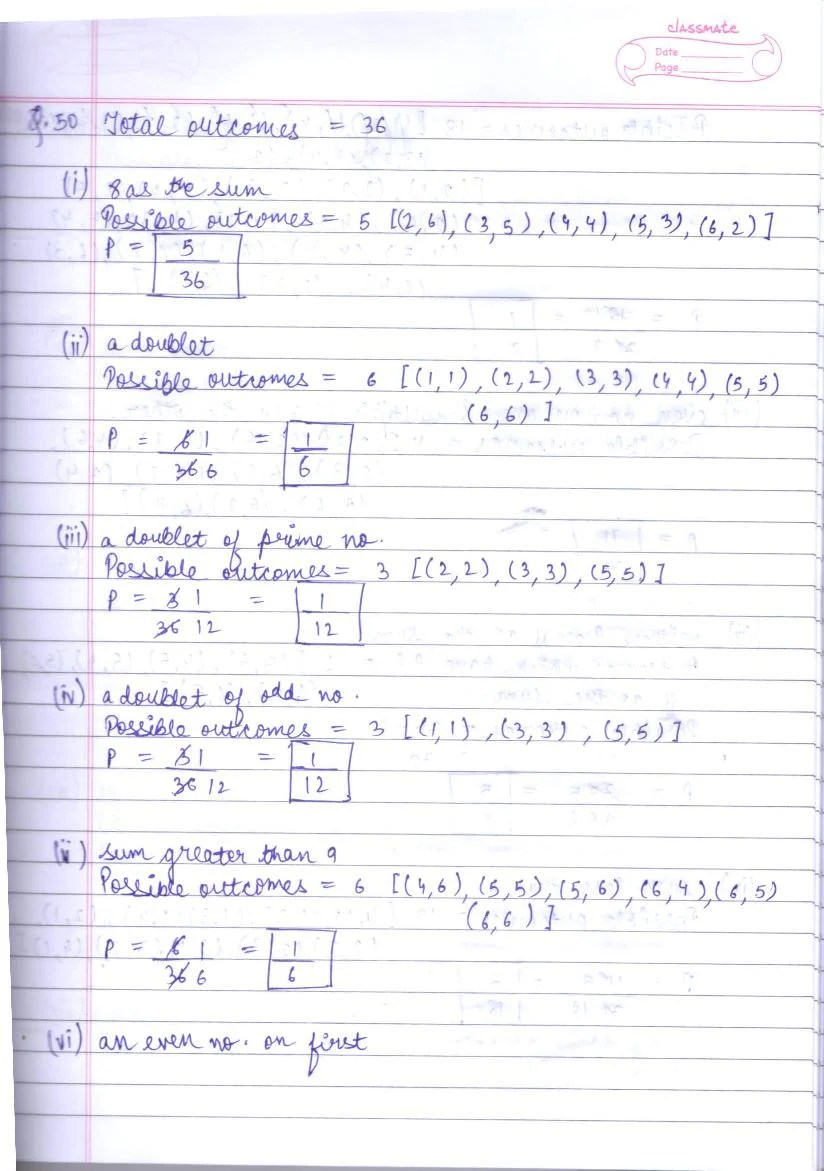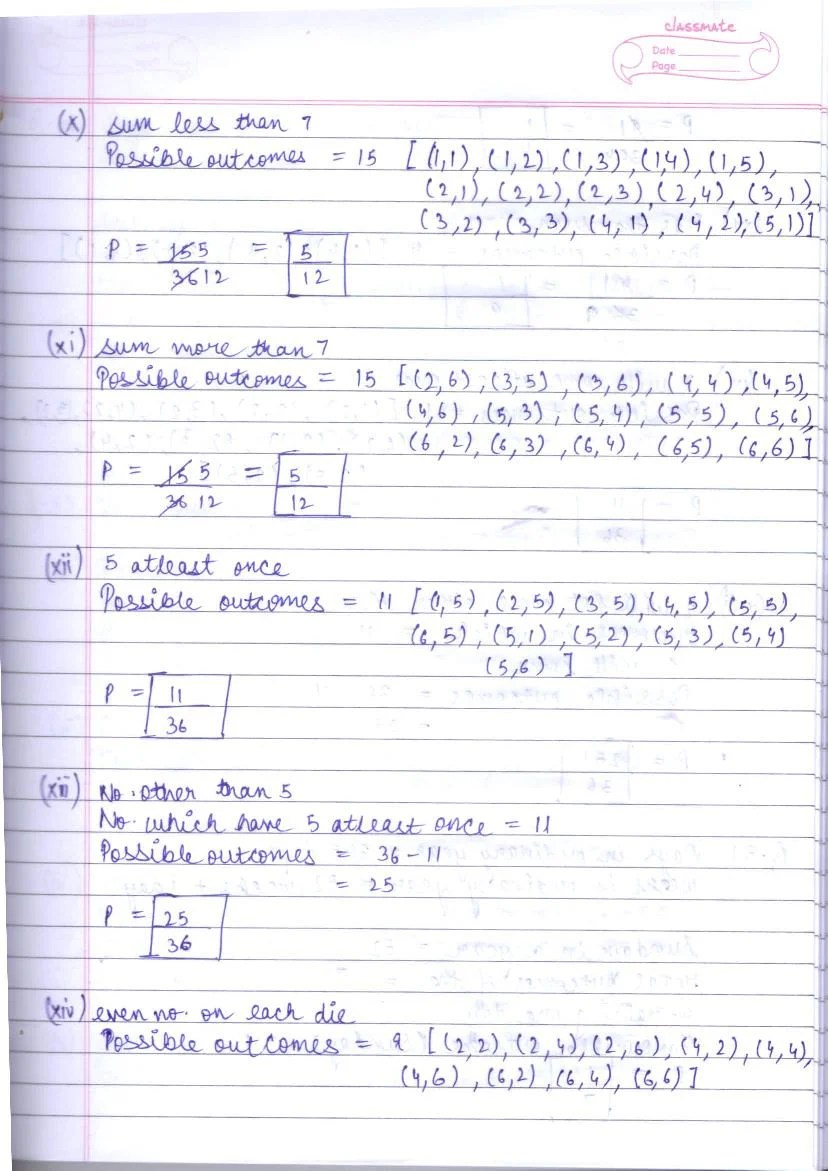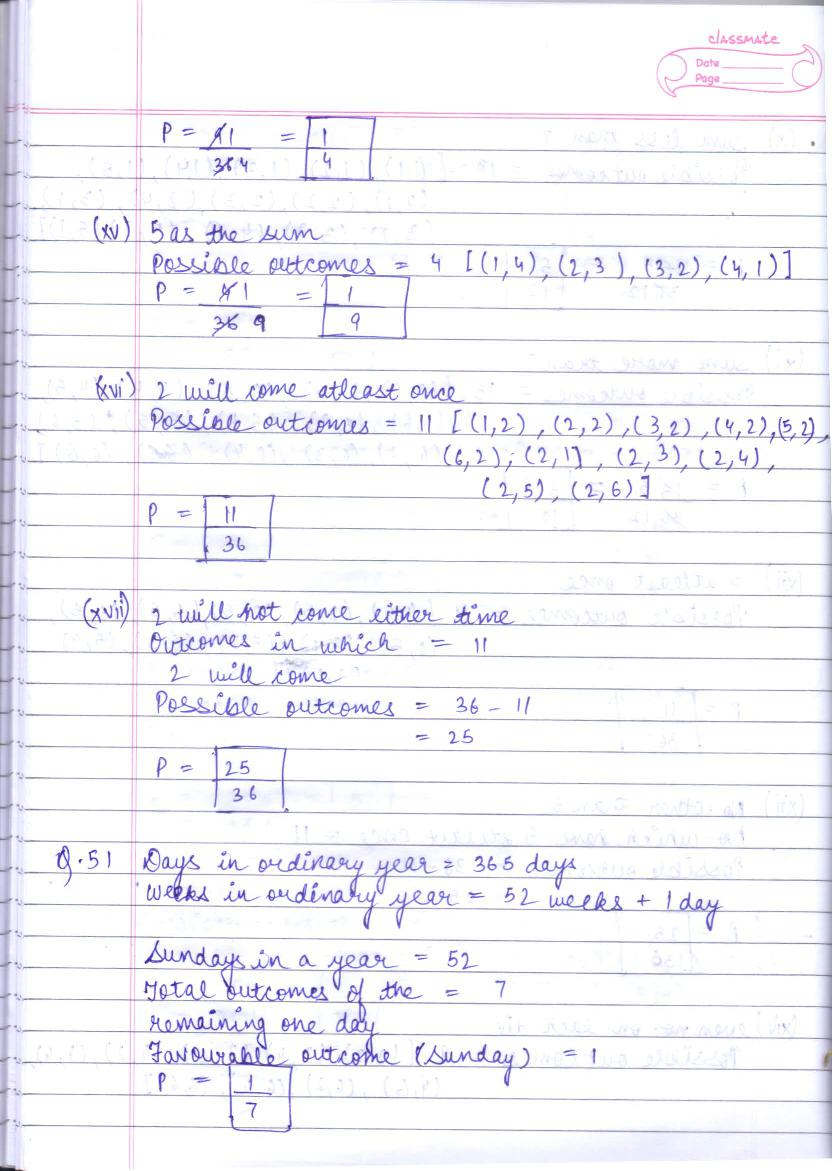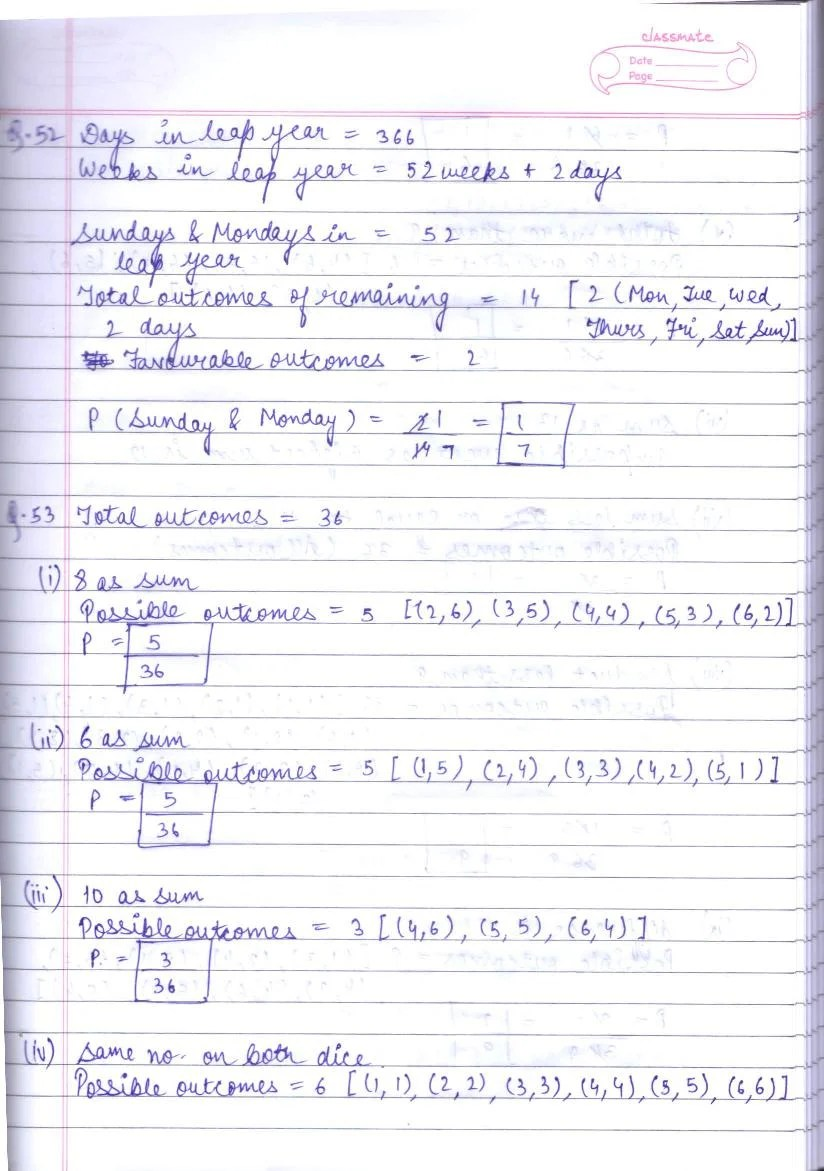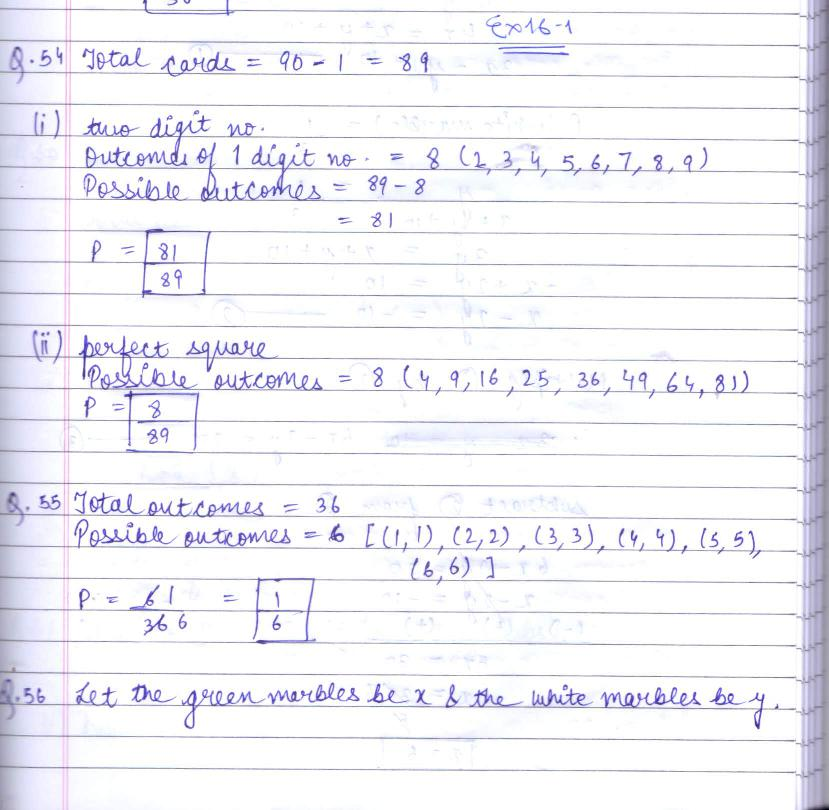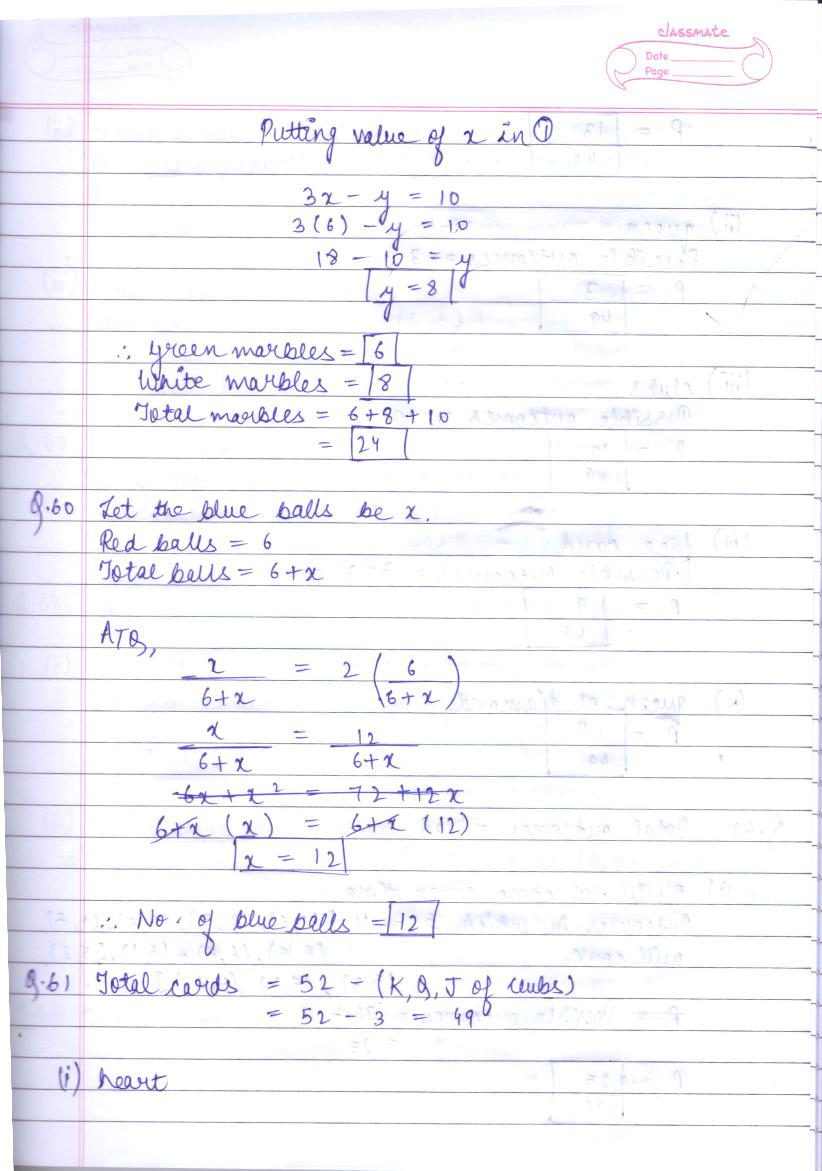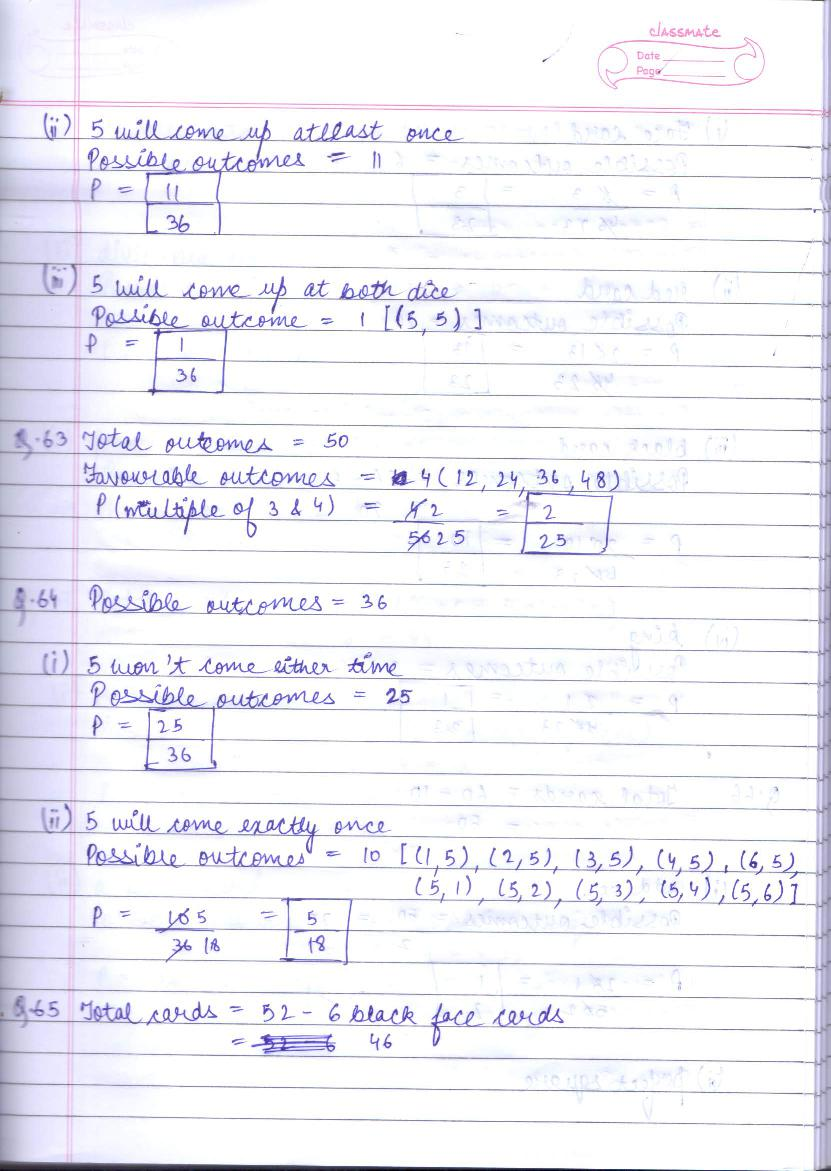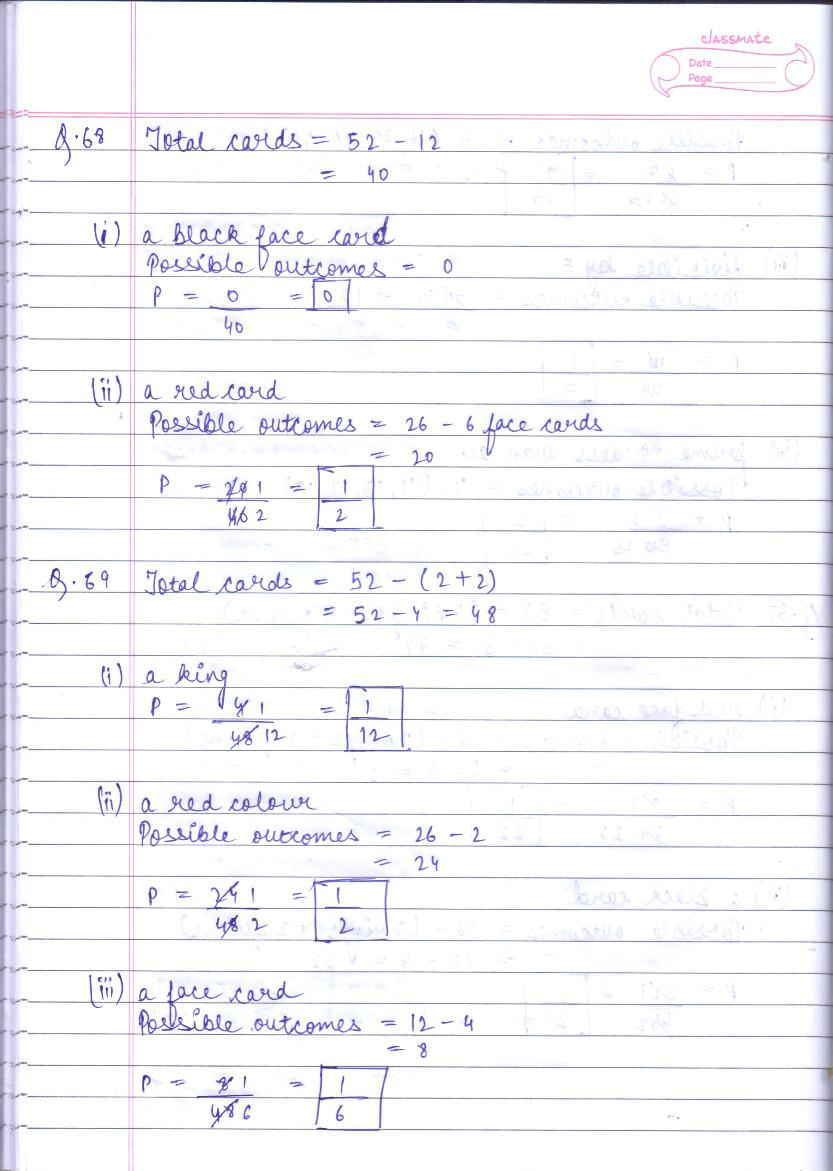# RD Sharma Solutions Class 10 Chapter 16 Probability Exercise 16.1

Here you can get free RD Sharma Solutions for Class 10 Maths Chapter 16 Probability Exercise 16.1. All RD Sharma Book Solutions are given here exercise wise for the chapter Probability. RD Sharma Solutions are helpful in the preparation of several school level, graduate and undergraduate level competitive exams. Practicing questions from RD Sharma Mathematics Solutions for Class 10 Chapter 16 Probability is proven to enhance your math skills.

 Class: Class 10th Chapter: Chapter 16 Exercise: Exercise 16.1 Name: Probability

## RD Sharma Solutions Class 10 Chapter 16 Probability Exercise 16.1

RD Sharma Class 10 Solutions Chapter 16 for Probability Exercise 16.1 are given below.

RD Sharma Solutions Class 10 Chapter 16 Probability Exercise 16.1